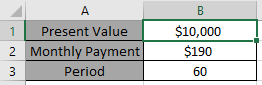# How to use the RATE Function in Excel

RATE function is used to find the Interest rate of the data set in Excel. It requires the following arguments to calculate the interest rate.
Syntax:

=RATE (nper, pmt, pv, [fv], [type], [guess])

nper: total no. of payment period.
pmt: amount paid each period.
pv - The present value of future payments must be entered as a negative number.
fv: [optional] due future value. Default is 0
type - [optional] When payments are due. Default is 0.

Let’s use it in an example to understand it.Use the formula

=RATE(B3,B2,-B1)*12Press Enter.The interest rate for the data set is 5%.
Hope you understood how to use RATE function to get the Interest rate of the data. Explore more articles on Accounting here. Please write your queries in the comment box below. We will help you.

Related Articles:

Simple interest formula in Excel

Excel IPMT Function

Calculate Profit margin percentage

Excel CUMIPMT Function

How to Use RATE Function in Excel

Popular Articles:

How to use the VLOOKUP Function in Excel

How to use the COUNTIF function in Excel 2016

How to Use SUMIF Function in Excel

Terms and Conditions of use

The applications/code on this site are distributed as is and without warranties or liability. In no event shall the owner of the copyrights, or the authors of the applications/code be liable for any loss of profit, any problems or any damage resulting from the use or evaluation of the applications/code.